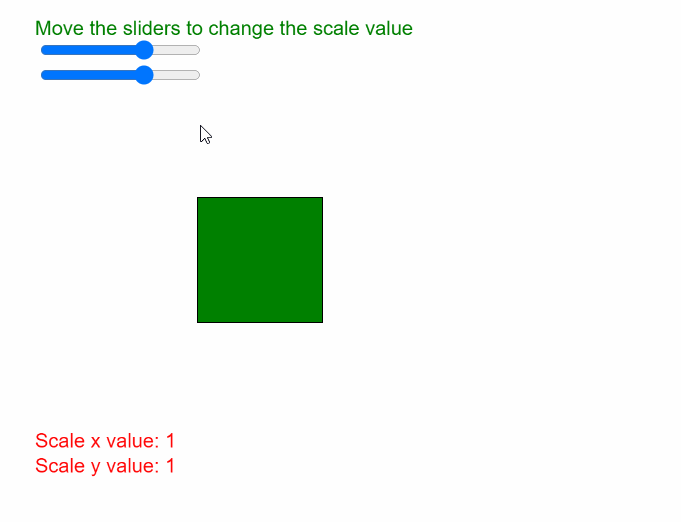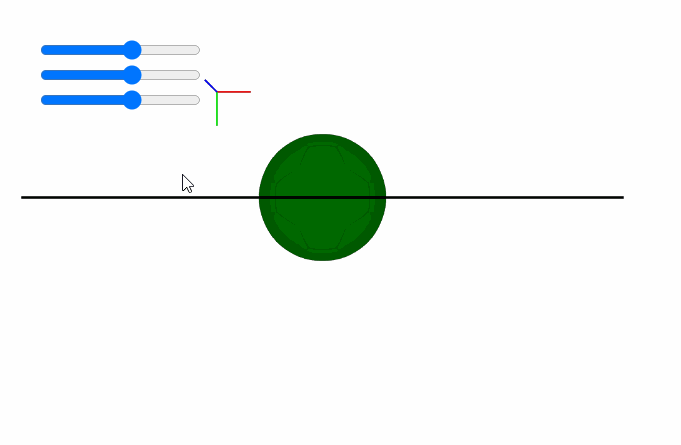GeeksforGeeks App
Open AppBrowser
Continue

## Related Articles

The scale() function in p5.js is used to increase or decrease the size of a shape or model by expanding or contracting its vertices. The scale values are specified as decimal percentages, that is, a scale value of “2.0” would increase the dimensions of the shape by 200%. Similarly, a negative of “-2.0” would decrease the dimensions of the shape by 200%

The objects always scale from their relative origin to the coordinate system. The z parameter of this function is only available in the WebGL mode for scaling across the z-axis.

As `scale()` is a transformation, every transformation that happens after one call multiplies the effect. If `scale()` is called within the `draw()` loop, then the transformation is reset when the loop begins again.

Syntax:

`scale( s, [y], [z] )`

OR

`scale( scales )`

Parameters: This function accepts four parameters as mentioned above and described below.

• s: It is a p5.Vector, Number or array of Numbers which define the percent to scale the object. If multiple arguments are given, it denotes the percent to scale the object in the x direction.
• y: It is a Number which denotes the percent to scale the object in the y direction. It is an optional parameter.
• z: It is a Number which denotes the percent to scale the object in the z direction. It is an optional parameter.
• scales: It is a p5.Vector or array of Numbers which specify per-axis percents to scale the object.

The program below illustrate the scale() function in p5.js:

Example 1:

 `function` `setup() {``  ``createCanvas(500, 400);``  ``textSize(16);`` ` `  ``scaleXslider = createSlider(-3, 3, 1, 0.1);``  ``scaleXslider.position(30, 30);`` ` `  ``scaleYslider = createSlider(-3, 3, 1, 0.1);``  ``scaleYslider.position(30, 50);``}`` ` `function` `draw() {``  ``clear();``  ``text(``"Move the sliders to change the scale value"``, 20, 20);``  ``fill(``"red"``);``  ``rect(150, 150, 100, 100);`` ` `  ``// Get the scale parameters``  ``let scaleXValue = scaleXslider.value();``  ``let scaleYValue = scaleYslider.value();`` ` `  ``text(``"Scale x value: "` `+ scaleXValue, 20, 350);``  ``text(``"Scale y value: "` `+ scaleYValue, 20, 370);`` ` `  ``// Set the scale according to properties``  ``scale(scaleXValue, scaleYValue);`` ` `  ``fill(``"green"``);``  ``rect(150, 150, 100, 100);``}`

Output:Example 2:

 `function` `preload() {``  ``ballObj = loadModel(``"models/ball.obj"``, ``true``);``}`` ` `function` `setup() {``  ``createCanvas(500, 300, WEBGL);`` ` `  ``scaleXslider = createSlider(-3, 3, 0.5, 0.1);``  ``scaleXslider.position(30, 30);`` ` `  ``scaleYslider = createSlider(-3, 3, 0.5, 0.1);``  ``scaleYslider.position(30, 50);`` ` `  ``scaleZslider = createSlider(-3, 3, 0.5, 0.1);``  ``scaleZslider.position(30, 70);`` ` `  ``debugMode();``}`` ` `function` `draw() {``  ``clear();``  ``noStroke();``  ``lights();``  ``orbitControl();`` ` `  ``// Get the scale parameters``  ``let scaleXValue = scaleXslider.value();``  ``let scaleYValue = scaleYslider.value();``  ``let scaleZValue = scaleZslider.value();`` ` `  ``// Set the scale according to properties``  ``scale(scaleXValue, scaleYValue, scaleZValue);`` ` `  ``fill(``"green"``)``  ``model(ballObj);``}`

Output:Reference: https://p5js.org/reference/#/p5/scale

My Personal Notes arrow_drop_up
Related Tutorials